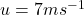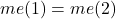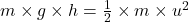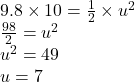## a 5kg bag falls a verticle height of 10m before hitting the ground. Ek=1/2mv^2 and Ep=m g h (assume g=9.8N/kg). Using these two

Question

a 5kg bag falls a verticle height of 10m before hitting the ground.
Ek=1/2mv^2 and Ep=m g h (assume g=9.8N/kg).
Using these two equations, calculate the speed the bag reaches before hitting the ground.

in progress 0
2 months 2021-07-23T12:51:26+00:00 1 Answers 4 views 0Explanation:

We know that when we don’t have air friction on a free fall the mechanical energy (I will symbololize it with ME) is equal everywhere. So we have:where me(1) is mechanical energy while on h=10m

and me(2) is mechanical energy while on the ground

Ek(1) + DynamicE(1) = Ek(2) + DynamicE(2)

Ek(1) is equal to zero since an object that has reached its max height has a speed equal to zero.

DynamicE(2) is equal to zero since it’s touching the ground

Using that info we havewe divide both sides of the equation with mass to make the math easier.